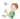## 抛硬币出现连续正面的概率计算

Mon, 4th November 2019Edit on GithubcoinprobabilityFibonacci

# 摘要

mathe于2008年7月引用百度知道中一个抛硬币的概率问题

shshsh_0510发现这个问题广义t阶Fibonacci数列 有关系, 其中不出现t次连续正面的概率为 $\frac{F_{n+2}^{(t)}}{2^n}$

# 具体内容

## 数值计算结果

$F_{102}^{(10)} = 1211700015849788251502892752696$ 至于那个分式 = 0.9558627713595783 于是投100次硬币出现连续10次以上的概率为1-0.9558627713595783=0.0441372286404217。

## 公式解分析

$|z^{t+1}|=d^{t+1}\le 2d-1\le |2z-1|$ 所以根据儒歇定理，我们知道$h(y)$在圆$|z|\le d$内部解的数目同方程$-2z+1=0$的数目相同。也就是在$|z|\lt 1$内方程$h(z)=0$只有一个解。而如果$|z|=1$满足$h(z)=0$,那么容易得到z=1,而且根的重数为1。

$u_1z_1^{-n}+u_2z_2^{-n}+...+u_{t}z_t^{-n}$

$k(z)=(z-z_1)(z-z_2)(z-z_3)...(z-z_t)$
$k'(z_s)=\prod_{h\ne s} (z_s-z_h)$
$h'(z_s)=(z-1)\prod_{h\ne s} (z_s-z_h)$

$u_s=\frac{z_s-1}{h'(z_s)}$
$h'(z_s)=(t+1)z_s^t-2=(t+1)(2-z_s^{-1})-2=2t-(t+1)z_s^{-1}$

$u_s=\frac{z_s-1}{2t-(t+1)z_s^{-1}}$

$r_t=z_1^{-1}$

$b(n)=\lfloor\frac{r_t^{-1}(1-r_t)}{2t-(t+1)r_t} r_t^{n}\rceil$

n b(n) $u_1r_t^n$
1 1 0.50222005921650437539314900083299
2 1 1.0039472560945626042296717505261
3 2 2.0069092711912102894563100627129
4 4 4.0118490272698787619203081600742
5 8 8.0197609571323822998698197638132
6 16 16.031651583188626895454838284701
7 32 32.047570227910457178387829251568
8 64 64.063690018678506437470207581223
9 128 128.06451002750246161567818042825
10 256 256.00334173386700758850659443979
11 512 511.75545016205159804636690872342
12 1023 1023.0086802648866917173406684459
13 2045 2045.0134132736788208304516651412
14 4088 4088.0199172761664313714470202193
15 8172 8172.0279855250629839809737322779

$\frac{r^{-1}(r-1)}{(t+1)r-2t}\lt\frac12$

$e(1)=1-\frac{r-1}{(t+1)r-2t}$,所以$0\lt e(1)\lt \frac12$

$b(n)=\lfloor\frac{r^{-1}(r-1)}{(t+1)r-2t}r^n\rceil$

$p(n)=1-\frac{\lfloor\frac{r-1}{(t+1)r-2t}r^{n+1}\rceil}{2^n}; r\gt 1; r^{t+1}-2r^t+1=0$

Github